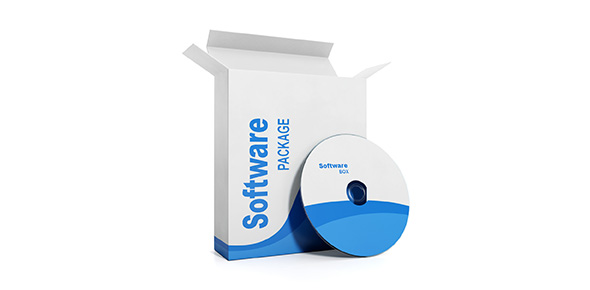# Basics Of Software Defined Radio Architectures And Building Blocks Of Sdr Waveform Development For Sdr

25 Questions | Total Attempts: 80SettingsCreate your own Quiz• 1.
What is the major drawback of Superheterodyne transceivers with respect to homodyne receivers?
• A.

I/Q imbalance

• B.

LO leakage

• C.

DC Offset

• D.

Difficult to integrate on-chip

• 2.
The transmit frequency is kept higher to
• A.

Be able to use smaller antenna size

• B.

• C.

Both of above

• D.

None of above

• 3.
Which of the following is a drawback of Homodyne transceivers:
• A.

I/Q imbalance component can be avoided by filtering.

• B.

Large size as compared to other architectures.

• C.

Difficult to integrate on-chip

• D.

DC offset errors interefere with signal of interest.

• 4.
Without using an IF frequncy, the desired signal is directly frequency downconverted to baseband / frequency up-converted to RF in
• A.

Superheterodyne transmitter/ Superheterodyne receiver

• B.

Superheterodyne receiver/ Superheterodyne transmitter

• C.

Homodyne receiver/ Homodyne transmitter

• D.

Homodyne transmitter/ Homodyne receiver

• 5.
If measure SNR is around 98 dB in an ADC. What is bit-resolution of ADC ?
• A.

16-bit

• B.

10-bit

• C.

12-bit

• D.

8-bit

• 6.
Which of the following is merit of out-of-band error
• A.

NMSE

• B.

ACPR

• C.

EVM

• D.

BER

• 7.
Numerically Controlled Oscillators is used in
• A.

Superheterodyne architecture

• B.

Digital-IF based Homodyne architecture

• C.

Homodyne architecture

• D.

Dual-conversion superheterodyne architecture

• 8.
Increase in fIF (IF frequency) in Superheterodyne transceivers is not related to
• A.

Capability of filtering the image signal.

• B.

Increase in constraint on DAC and ADC.

• C.

Increase in clock jitter.

• D.

Increase in I/Q imbalance component power

• 9.
Digital-IF based Homodyne transmitter require
• A.

Numerically Controlled Oscillators

• B.

Two LOs

• C.

Two bandpass filters.

• D.

More than two LOs and Oscillators.

• 10.
In an analog quadrature modulator, frequency of input data is 30 MHz and LO frequency is1.8 GHz. What would be frequency of output RF?
• A.

1.8 GHz

• B.

1.86 GHz

• C.

1.83 GHz

• D.

None of the above

• 11.
This is not a benefit of oversampling:
• A.

Improved bit-resolution of ADC

• B.

Increase in SNR.

• C.

Avoid aliasing

• D.

Decrease in I/Q imbalance signal power.

• 12.
Which of the following is not true regarding software-defined-radios
• A.

Functions are digitally defined

• B.

Reconfigurable

• C.

No hardware is required at all

• D.

Algorithms should be updated according to the digital platform

• 13.
Pulse shaping is applied on the digitally modulated signal to
• A.

Enhance signal quality

• B.

• C.

Contain the signal information in smaller bandwidth

• D.

Remove the need for power amplifier

• 14.
Which of the following are the limitations in achieving (completely digital) ideal software define radios
• A.

Baseband Processing

• B.

Local Oscillators, due to phase noise

• C.

Sampling speed of A/D and D/A, which is sufficient to cover transmission frequency

• D.

Data encoding which consumes digital resources

• 15.
Please consider following sentences (i) Rectangular signal in time domain corresponds to a SINC signal in frequency domain. (ii) Rectangular signal in frequency domain corresponds to a SINC signal in time domain.
• A.

Only (i) is true

• B.

Only (ii) is true

• C.

Both (i) and (ii) are wrong

• D.

Both (i) and (ii) are correct

• 16.
Select the correct statement
• A.

SNR is lower than the SNDR

• B.

SINAD is lower than the SNDR

• C.

SINAD is lower than the SNR

• D.

SNR is lower than the SINAD

• 17.
Which of the following are most suitable for computation heavy applications
• A.

Digital signal processor

• B.

Graphics processing units

• C.

General-purpose microprocessors

• D.

Field programmable gate arrays

• 18.
Highest frequency in the discrete domain is given by
• A.

Angular frequency π, frequency 0.5.

• B.

Angular frequency π, frequency 0.75.

• C.

Angular frequency 4π, frequency 0.5.

• D.

Angular frequency π, frequency 1.

• 19.
We need to apply N- point DFT for 16382 samples, which of the following is the most suitable value of N to be implemented in a DSP
• A.

16384

• B.

10000

• C.

15000

• D.

16380

• 20.
Applying windowing techniques in time domain waveform results in
• A.

• B.

Better attenuation of side lobes

• C.

Less spectrum leakage

• D.

All of the above

• 21.
Under-sampling methods allow utilizing lower sampling rates than the highest frequency of the incoming signal for
• A.

Pass-band signal

• B.

Base-band signal

• C.

Both of above

• D.

None of above

• 22.
For band-pass sampling
• A.

Lower ADC bandwidth is required as compared to baseband sampling technique.

• B.

The sampling rate of ADC is always higher than the baseband sampling technique.

• C.

Both of above

• D.

None of above

• 23.
For measuring time domain performance and frequency domain performance respectively, we require
• A.

Oscilloscope and Spectrum analyzer respectively.

• B.

Spectrum analyzer and Oscilloscope respectively.

• C.

Oscilloscope only.

• D.

Spectrum analyzer only.

• 24.
If the IF frequency in a transmitter is equal to 0 then the architecture of the transmitter is called
• A.

Superheterodyne / Digital-IF

• B.

Homodyne / Direct Conversion.

• C.

Superheterodyne / Direct Conversion

• D.

Homodyne / Digital-IF

• 25.
What to do you understand by Digital IF
• A.

IF Shift using analog component

• B.

IF Shift in baseband

• C.

Both a and b

• D.

None of the above.

Related TopicsBack to top
×

Wait!
Here's an interesting quiz for you.